Courses

# Test: Analog Electronics - 1

## 25 Questions MCQ Test Analog Circuits | Test: Analog Electronics - 1

Description
This mock test of Test: Analog Electronics - 1 for Electrical Engineering (EE) helps you for every Electrical Engineering (EE) entrance exam. This contains 25 Multiple Choice Questions for Electrical Engineering (EE) Test: Analog Electronics - 1 (mcq) to study with solutions a complete question bank. The solved questions answers in this Test: Analog Electronics - 1 quiz give you a good mix of easy questions and tough questions. Electrical Engineering (EE) students definitely take this Test: Analog Electronics - 1 exercise for a better result in the exam. You can find other Test: Analog Electronics - 1 extra questions, long questions & short questions for Electrical Engineering (EE) on EduRev as well by searching above.
QUESTION: 1

### To prevent a DC return between source and load, it is necessary to use

Solution:

Capacitor offers infinite impedance to DC.

QUESTION: 2

### For a base current of 10 μA, what is the value of collector current in common emitter if βdc = 100

Solution:

IC = 10 x 100 μA = 1 mA.

QUESTION: 3

### Which of the following oscillators is suitable for frequencies in the range of mega hertz?

Solution:

Only LC oscillators are suitable for MHz range.

QUESTION: 4

If the input to the ideal comparator shown in the figure is a sinusoidal signal of 8 V (peak to peak) without any DC component, then the output of the comparator has a duty cycle of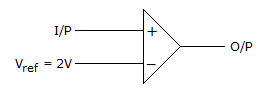Solution: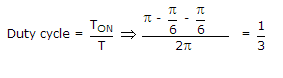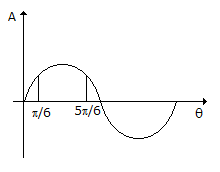QUESTION: 5

A half wave diode circuit using ideal diode has an input voltage 20 sin ωt volts. Then average and rms values of output voltage are

Solution: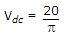and VC = 0.5 x 20 = 10 V.

QUESTION: 6

An RC coupled amplifier has an open loop gain of 200 and a lower cutoff frequency of 50 Hz. If negative feedback with β = 0.1 is used, the lower cut off frequency will be

Solution:

New lower cutoff frequency =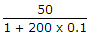.

QUESTION: 7

In figure v1 = 8 V and v2 = 4 V. Which diode will conduct?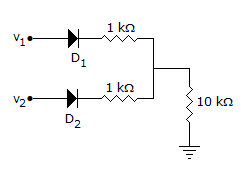Solution:

D1 will conduct and the output voltage will be about 7 V. Therefore D2 will be reverse biased and will not conduct.

QUESTION: 8

The load impedance ZL of a CE amplifier has R and L in series. The phase difference between output and input will be

Solution:

It is 180º for purely resistive load and between 180º and 270ºor R-L load.

QUESTION: 9

Gain of the amplifier is 'A'. Then the I/P impedance and O/P impedance of the closed loop amplifier shown below would be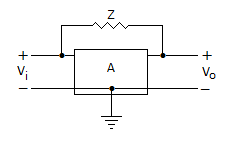Solution:

By using Miller theorem circuit can be redrawn as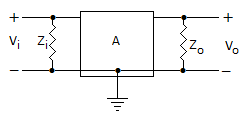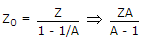.

QUESTION: 10

If an amplifier with gain of - 1000 and feedback factor β = - 0.1 had a gain change of 20% due to temperature, the change in gain of the feedback amplifier would be

Solution: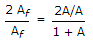As we know, Gain with feedback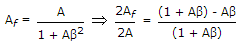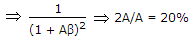A = - 1000, β = -0.1 .

QUESTION: 11

In figure The minimum and maximum load currents are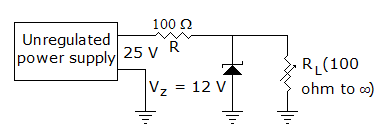Solution:

When RL = ∞, IL = 0,

When RL = 100 Ω,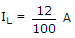or 120 mA.

QUESTION: 12

In figure, VEB = 0.6 V, β = 99. Then VC and IC areSolution: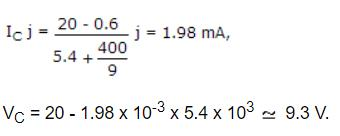QUESTION: 13

The input impedance of op-amp circuit of figure is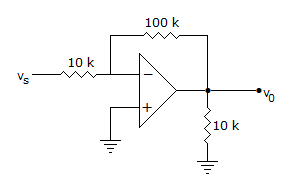Solution:

Due to the presence of virtual ground at input, the resistance in the series path of input of inverting amplifier is input impedance.

QUESTION: 14

In a BJT circuit a pnp transistor is replaced by npn transistor. To analyse the new circuit

Solution:

All voltages and currents have reverse polarity.

QUESTION: 15

To protect the diodes in a rectifier and capacitor input filter circuit it is necessary to use

Solution:

Resistor reduces surge current.

QUESTION: 16

The output V0 in figure is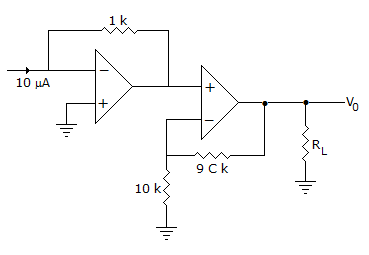Solution:

Input to non-inverting op-amp is -10 x 10-6 x 103 = -10 mV.

Therefore output =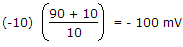= -100 mV.

QUESTION: 17

In a CE amplifier the input impedance is equal to the ratio of

Solution:

Input is applied to base with emitter grounded. The input impedance is the ratio of ac base voltage to ac base current.

QUESTION: 18

For a system to work, as oscillator the total phase shift of the loop gain must be equal to

Solution:

Gain of system with + ve feedback =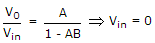for oscillation

but V0 ≠ 0

so, that 1 - AB = 0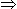AB = 1 ∠0ºor 360º.

QUESTION: 19

An amplifier has a large ac input signal. The clipping occurs on both the peaks. The output voltage will be nearly a

Solution:

When a sinusoidal voltage is clipped on both sides it resembles a square wave.

QUESTION: 20

The transistor of following figure in Si diode with a base current of 40 μA and ICBO = 0, if VBB = 6V, RE = 2 kΩ and β = 90, IBQ = 20 μA then RB =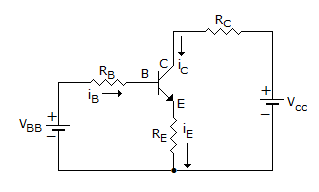Solution: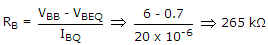.

QUESTION: 21

In the amplifier circuit of figure hfe = 100 and hie = 1000 Ω. The voltage gain of amplifier is about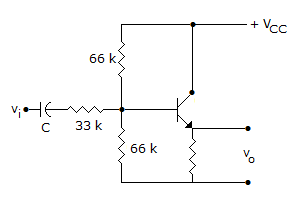Solution: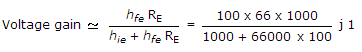.

QUESTION: 22

The efficiency of a full wave rectifier using centre tapped transformer is twice that in full wave bridge rectifier.

Solution:

Efficiency of full wave rectifier with centre tapped transformer is slightly higher than that of bridge rectifier.

QUESTION: 23

Assertion (A): CE amplifier is the most widely used BJT amplifier

Reason (R): CE amplifier has zero phase difference between input and output

Solution:

There is 180� phase difference between input and output.

QUESTION: 24

Assertion (A): For large signal variations an amplifier circuit has to be analysed graphically

Reason (R): The output characteristics of a transistor is nonlinear.

Solution:

Since the characteristics is non-linear, graphical analysis is needed.

QUESTION: 25

Negative feedback reduces noise originating at the amplifier input.

Solution:

It has no effect on noise originating at amplifier input.